Courses

# Physics General - General Science UPSC Notes | EduRev

## UPSC : Physics General - General Science UPSC Notes | EduRev

The document Physics General - General Science UPSC Notes | EduRev is a part of the UPSC Course Science & Technology for UPSC CSE.
All you need of UPSC at this link: UPSC

Motion

Motion is the state of a physical body during the time in which it changes its position with respect to a fixed point. Motion can be in a straight line (linear motion), along a curved line (angular motion) along a circle (circular motion), uniform, or non-uniform.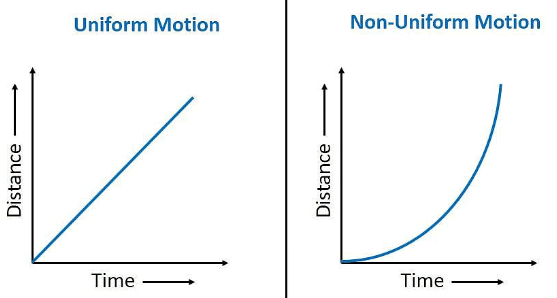Distance - Time Graph

Speed

The speed of a moving body is the rate at which it covers distance, the distance it covers per unit of time.

S.I Unit of Speed = Metre/Sec.

Velocity

The distance covered by an object in a specified direction in the unit time interval is called velocity.

S.I Unit of Velocity = Metre/Sec.

The difference between speed and velocity is that speed refers only to the distance covered by a moving object whereas velocity takes into account the direction also.

Acceleration

The velocity of a body changes due to change in its speed or direction or both. The rate of change of velocity of a body is called its acceleration.

S.I. Unit of Acceleration = Metre/Sec2.

Circular Motion

(i) 1 radian = 360°/ (2π) = 57.296°

(ii) One full revolution about a circular path involves a change of 2π radians in the angular position of a body. If a body is moving in a circular path, it will reach its original position after one rotation. Thus the displacement in one rotation will be zero but the distance traveled will be equal to the circumference.

The rate of angular displacement of a body moving along a circular path is angular velocity. It is measured by the angle swept over in unit time by the line joining the object to the axis of rotation.

Inertia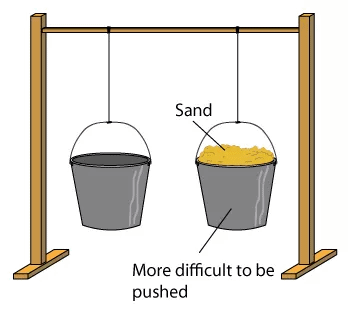Inertia and amount of substance. The property of all material bodies due to which they naturally tend to continue in a state of rest or of uniform linear motion. The inertia possessed by a body is measured as its mass. It was Galileo who first discovered the law of inertia.

Scalars and Vectors

• Scalar - A scalar is a quantity that has only magnitude. Examples:-Mass, time, density, temperature, etc.
• Vector - A vector is a quantity that has magnitude as well as direction. Examples: linear displacement, linear velocity, force, etc. A vector is represented by a straight line with a suitable scale. An arrow on the line represents the direction of the vector while the length of this line is proportional to the magnitude of the vector quantity (i.e., represents the magnitude).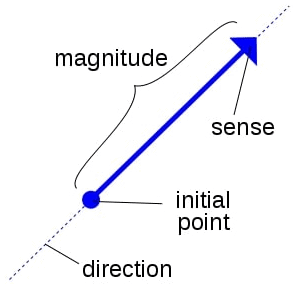A vector Representation

Force

Force is the action that alters or tends to alter the state of rest or uniform linear motion of a body. It is a vector quantity and is represented by F. When a man Kicks a ball, his foot exerts a force on the ball causing it to move.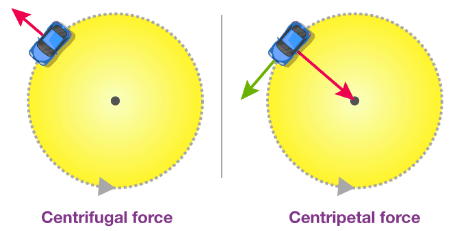Centrifugal vs Centripetal force

Centripetal Force

For a body to move in a circle there must be a force on it directed towards the center. This is called the centripetal force and is necessary to produce a continuous change of direction in a circular motion.

In the case of the moon, the gravitational force between the earth and the moon acts as the centripetal force. When a stone tied at on end of a string is whirled in a circle, the pull in the string provides the centripetal force.

Centrifugal Force

This is a pseudo force and it describes the motion of a particle for an accelerated (or rotating) observer.

Friction

Friction is the force opposing relative motion between two surfaces that are in contact with each other. Even when there is no relative motion, frictional forces exist between the surfaces.

A frictionless world would not at all be convenient. It would be difficult to sit on a chair without friction. It helps in supporting ladders against a wall, helps to start cars and trains. The brakes of a car or any moving device depend on friction.

In machines friction wastes energy. As one surface of anybody is moved over another, some energy is used up in overcoming the friction (dissipated into heat energy). Wear and tear of machinery is a result of excessive friction. A motor car engine will seize if it runs short of oil. The pistons and cylinders get so hot on account of excessive friction that they become jammed together.

Linear Momentum

This is a measure of the linear motion possessed by a body. It is a vector quantity.

Momentum = (mass of the body) x (linear velocity)

It is measured in kilogram-meter per second (kgm/s). Its direction is the same as the linear velocity of the body. Impulse: The change of linear momentum produced by a force in an accelerated or decelerated body per unit time is the impulse.

Newton's Laws of Motion
Newton’s First Law (Law of inertia)

Everybody continues in its state of rest or of uniform motion in a straight line unless compelled by an external force to act otherwise.”

Several phenomena illustrate this law. If a moving vehicle comes to a sudden stop, a passenger inside it jerks forward if he is not careful; this happens because though his feet remain in contact with the floor of the vehicle and come to rest suddenly, the upper part of the person’s body retains the forward movement.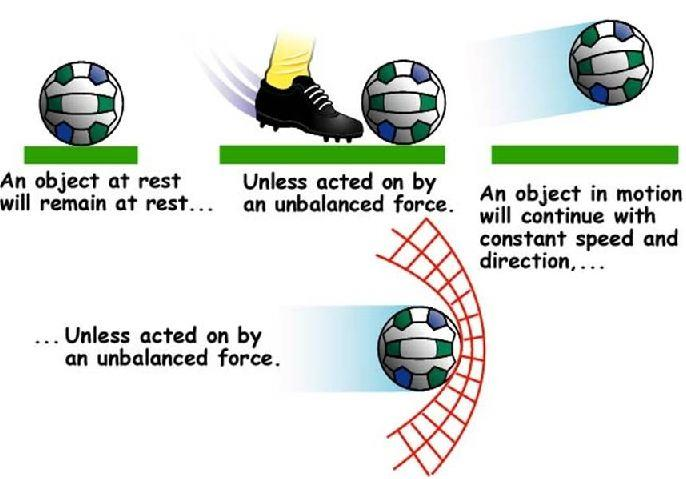Example of Newton's First law of motionRemoving dust from a carpet by beating it, running fast before a long jump, a small hole forming in a windowpane on a bullet being fired at it, etc.

Newton’s Second Law

“The rate of change of momentum of a body is proportional to the applied force and takes place in the direction in which the force acts.”

Mathematically, Newton’s second law is stated as, F = ma, where F = force, m = mass, and a = acceleration. Newton is the S.I. unit of the magnitude of the force.
One newton is the force that imparts an acceleration of one meter per second to a mass of one kilogram. It is represented by N. The second law comes into play when brakes are applied to a vehicle, and a large decelerating force is created to bring the vehicle to a stop. It also explains why a glass bottle dropped in the sand would not break: the yielding sand permits a lower deceleration than a hard floor.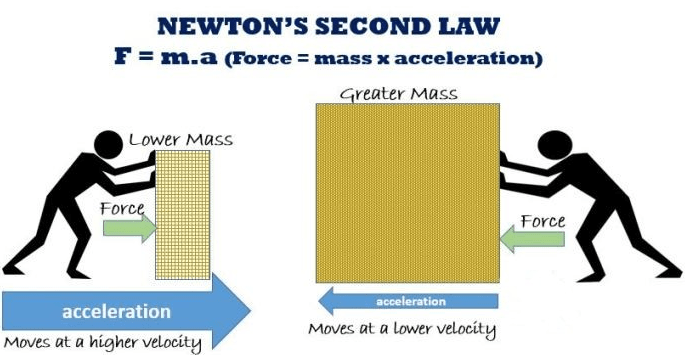Newton's Second law

Newton’s Third Law

This law states that “To every action, there is an equal and opposite reaction.”

If body A exerts a force on body B, then B exerts an equal and opposite force on A along the same line of action. If we fire a gun, the forward thrust of the projectile is matched by the backward thrust—the ‘kick’ or recoil of the gun. When we push against the wall with our hand, the wall exerts an equal amount of force on our hand.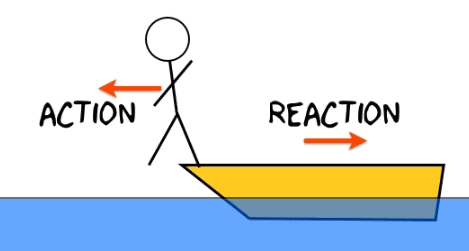Newtons' Third Law

Pressure

Pressure is defined as a force acting per unit area. The SI unit of pressure is Newton per square meter or Pascal (Pa).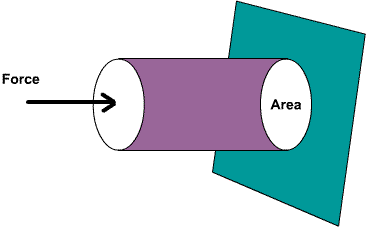Pressure

Zero Pressure

Zero pressure is the pressure in a given region of space when all the fluid, which is the source of pressure, has been removed from that region.
The perfect vacuum has zero pressure. Since the moon does not have any atmosphere, the pressurize zero. Interplanetary space also has zero pressure.

Gauge Pressure

The pressure measured with respect to the atmospheric pressure.

Absolute Pressure

The pressure measured with respect to zero pressure.

Atmospheric Pressure

This is the pressure due to the weight of the gases which make up the atmosphere around us. At a given place, the atmospheric pressure is due to the weight of the column of air above the area of application (up to the ‘top’ of the earth’s atmosphere). At sea-level, the height of the air column, and hence the atmospheric pressure, is maximum. At any height above sea-level, whether on a mountain or in an airplane, the air column, and hence the atmospheric pressure, is lesser than at sea-level.

One atmosphere is the pressure which supports a column of mercury 760 mm high at 0°C at sea-level and latitude 45°.1 atm = 101325 Pa. An average-sized man having a surface area of 2m2 will have a total thrust acting over his body of something in the region of 20t or 200kN. He is not of course, conscious of this enormous load, since his blood exerts a pressure slightly greater than the atmospheric pressure, and so a balance is more than effected. At high altitudes, where the pressure of the air is less, nose-bleeding may occur owing to the excess pressure of the blood. For every 10.3 m (approx. 10 m in seawater) a diver descends, the pressure on his body increases by one atmosphere. It is dangerous to stay longer at depths, since, as a result of the high pressure, an excess of nitrogen dissolves in the blood, and on return to the surface nitrogen bubbles form in the blood. Such a condition causes severe pain or even death.

The danger to health from the painful diver’s bends as this condition is called is greatly reduced if a mixture of 8 percent oxygen and 9.2 percent helium is used in gas cylinders. In contrast with the problems encountered by the diver, the crew and passengers in aircraft flying at high altitude would experience difficulty in breathing and consequent danger owing to less atmospheric pressure. The problem is overcome by “pressurizing” the aircraft. All openings are sealed, and a normal atmospheric pressure is maintained inside by the use of air pumps.

Facts To Be Remembered

• The definition and derivation of Force comes from the Newtons Second Law of Motion.
• Launching of rocket vehicles and jet planes follow the Newton’s Third Law of Motion.
• One gram weight is equal to 980 dynes.
• In C.G.S. (Centi-Gram-Second) system, the unit of Force is Dyne and in S.I. or MKS (Metre-Kilo-Second) system, the Newton.
• If a body is allowed to fall from the window of a moving train, the body will fall on the ground after following a parabolic path.
• A hunter should aim his gun to hit the target a little higher than the target. Because the bullet moves on a parabolic path. Similarly, projectile has the parabolic path.
• If a piece of ice is in a cylinder containing water, and ice melts completely, the level of water remains unchanged (According to Archimedes’ Principle).
• Hydrometer is an instrument for measuring relative density of liquids. The deeper the hydrometer floats in the liquid, the smaller is the relative density of the liquid. Density of a substance is the mass of a unit volume of that substance.

Facts To Be Remembered

• C.G.S. System- In this system the unit of length is the centimeter, the unit of mass is the gram and the unit of time is the second. It is called French System.
• F.P.S. System- In this system the unit of length is the foot, the unit of mass is the pound and the unit of time is the second. It is called British System of Unit.
• Chalk- It is a mixture of a number of substances, containing a large amount of calcium carbonate.
• Lime- It is a compound having its chemical name as calcium carbonate.
• Rest- A body is said to be at rest when it does not change its position with respect to the surrounding objects.
• Motion- A body is said to be in motion when it changes its position with respect the surrounding objects.
• Speed- It is the rate at which a body moves in any particular direction.
• Velocity- It is the rate at which a body moves in one particular direction.
• Power- It is the rate of doing work, and it indicates the rate at which work is done.
• Energy- Energy of a body is defined as the capacity to do work, and it indicates the total quantity of work that a body is capable of doing.
• Uniform velocity- If a body covers equal distance in equal intervals of time, it is said to move with a uniform velocity.
• Variable velocity- If a body covers unequal distances in equal intervals of time, or equal distances in unequal intervals of time. It is said to move with a variable velocity.
• Velocity-It is the rate at which a body moves in one direction.
• Acceleration- It is the rate of change of velocity of a body.
Offer running on EduRev: Apply code STAYHOME200 to get INR 200 off on our premium plan EduRev Infinity!

## Science & Technology for UPSC CSE

47 videos|159 docs|231 tests

,

,

,

,

,

,

,

,

,

,

,

,

,

,

,

,

,

,

,

,

,

;